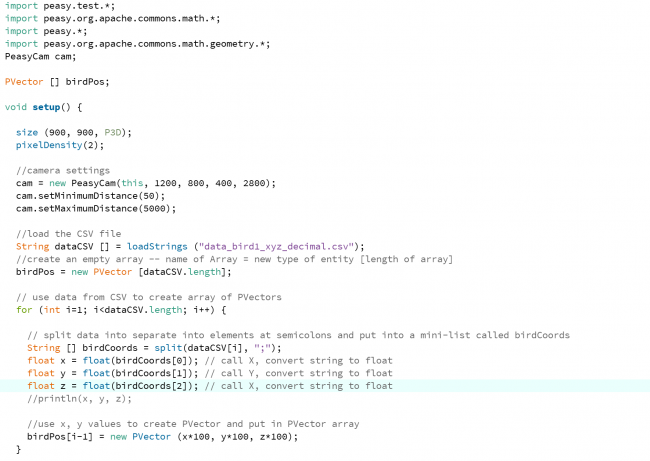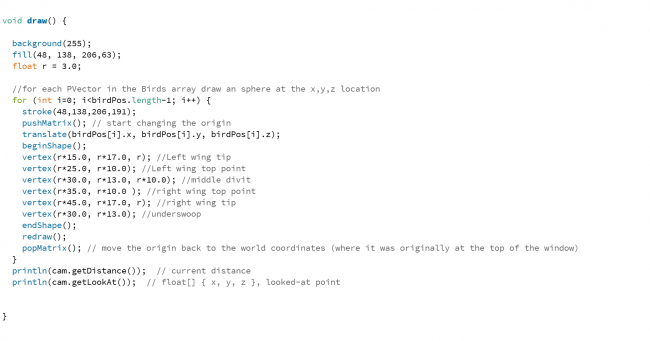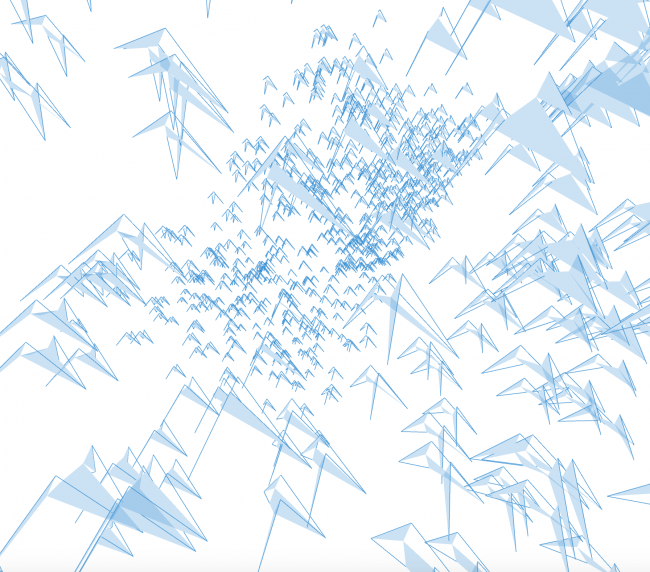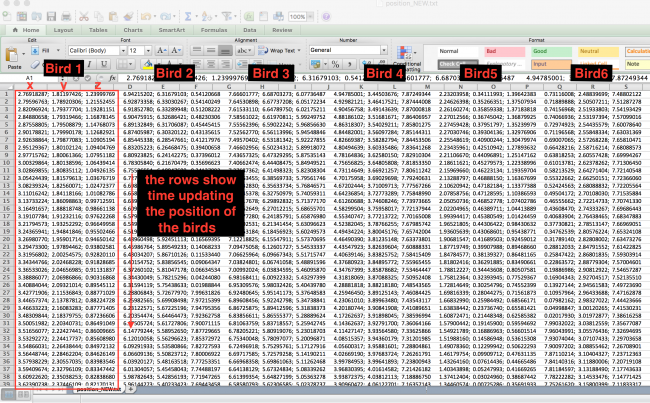#### Howdy, Stranger!

We are about to switch to a new forum software. Until then we have removed the registration on this forum.

# Is there a list of file types that are compatible to import into Processing ?

edited September 2016

Hi,

I am looking to export the data from 3D simulation coded in Python and import the data into Processing for a visualization. Is there a list of compatible file types or can someone recommend a format to use?

Many thanks! Janet

Tagged:

• What exact data are you talking about? Is this data you're creating, or is it a certain type of file, or something else?

• Hi Kevin,

So I have come to two conclusions regarding the bird flocking program that I am working on.

1) python mode in processing

In this case I would take the base code and run it first then I would make additions.

This option would allow for real time visual effects like adding more birds into the simulation with a mouse click etc.

2) import a CVS file or comparable data file into processing and script around it.

This would mean that the data from the initial simulation would be static and the code that I would write would purely a visualization of the data. Perhaps a benefit of this is that it would be faster to run considering the data is just being imported and the visualization is a separate code.

At this point I don't know which one would be a better option but in general I would like to learn how to realize data visualization in processing so this seems like a good opportunity to try it out.

So my question is: what is the best format for the simulated data? CVS?

Best, Janet

• Is there a reason you're restricting yourself to python mode?

Do you mean a CSV file? Sure, you can load that into your Processing code.

But I'm not sure what you mean by script around it, and I'm not sure what you mean by "better".

How are you creating the CSV file in the first place?

Either approach is equally fine. It really depends on what you're trying to do. Do you have an existing simulation that outputs CSV files? If so then it would definitely be possible to write Processing code that visualizes those files. Or are you trying to write the simulation in Processing? That's also very doable! Neither one is really "better" than the other. Which one you choose really depends on your goals.

I would get something working first and then worry about speed later. It might be that the speed of the simulation doesn't really matter, especially if your end goal is to create videos or animated gifs of the simulation, which can be sped up later.

• What is "the simulated data" -- it sounds like it is a time series of points or vectors for birds?

Try loadTable. Here is an additional example of its use by Daniel Shiffman.

If on the other hand you are already exporting JSON, XML, or something else, just import that. See some of the possibilities in the Processing reference under Input > Files

• Thank you for your comments! The simulated data is in the form of .txt files.

Right now I have three txt files which are the x, y, and z components of the bird movements.

the data points are floats.

something like

1) set up scene
2) define variable
3) import data file 1 as x coordinates
import data file 2 as y coordinates
import data file 3 as z coordinates
4) update the data points to show the simulation

--

does this make sense ?

``````void setup() {
size(200,100);
noLoop();
}

void draw() {

//Creating file of data
String words = "1.0 2.2 3.3 4.4 5.5 6.6 7.7 8.8 9.9 10.0 20.5 21.6 22.7 23.8 24.9 25.0";
String[] list = split(words, ' ');

// Writes the strings to a file, each on a separate line
saveStrings("numbers.txt", list);

println("there are " + lines.length + " lines");

float[] numb = float(lines);

for (int i = 0; i < numb.length; i++) {
println(numb[i]);
}
}
``````

If you are generating the data yourself, you should consider saving all the position (aka x,y,z data) in a single data file. CSV format would be convenient as you could use loadTable to manage your data. Using PVector objects in and an ArrayList will provide you ways to manage multiple points and to access PVector standard functions (check https://processing.org/reference/PVector.html) that might be handy for your application. I hope this helps,

Kf

• Cool, thank you!

Yes, I am generating the data myself. I have the x,y,z coordinates for each of the 500 birds, for a total of 1500 CSV files.

I am trying to create a visualization where those x,y,z coordinates are the center of each bird.

Does that make sense ?

• do you really mean "a total of 1500 CSV files"

why not one file, 500 lines, 3 values on each line?

• @JRafner -- I was also unclear based on your question:

Are you trying to create your Processing sketch in Python Mode, or in default Java Mode? Note that @kfrajer 's example is written for default Java, not Python.

• sorry for the slow response, It took a lot of time to get the data output correct.

I started with just one CSV file for one bird [xyz in three columns, time in 800 rows] and I got an output that I am pretty happy with, however it is static [i.e. all the birds appear at once instead of being animated] Do you have any suggestions ?

here is the code, it is written in the default Javaand here is a screen shot from the simulation• Please, do not post screenshots of your code. Instead copy and paste your code. It allows us to reproduce any problems and to make it searchable in case other ppl in the community happen to be working in something similar

Do you have any suggestions ?

Yes, you are loading all bird positions in draw. If you want to see action, you need to draw each positions one by one (instead of all of them). You need to remember draw executes at 60 fps.

Kf

• edited October 2016 Answer ✓

Squinting at that code, you could define a bird PShape in setup and just call shape () with the PShape in draw rather than defining the exact same geometry 800 times, 60 times a second.

Stroke can go outside the loop.

No need for redraw.

Using println a lot can cause trouble. Will definitely slow things down.

• sorry :) here attached is the code. I took some of your suggestions and am still working on creating the PShape in the set up, but still don't fully understand how to draw each of the positions one by one. Can anyone elaborate on that? Or perhaps direct me to an example? Thanks a lot for all the help -J

(mangled code deleted. reposted below)

• ``````import peasy.test.*;
import peasy.org.apache.commons.math.*;
import peasy.*;
import peasy.org.apache.commons.math.geometry.*;
PeasyCam cam;

PVector [] birdPos;
PVector [] birdTxtPos;

void setup() {

size (900, 900, P3D);
pixelDensity(2);

//camera settings
cam = new PeasyCam(this, 1200, 800, 400, 2800);
cam.setMinimumDistance(50);
cam.setMaximumDistance(5000);

String dataTXT [] = loadStrings ("position_NEW_sml.txt");

// use data from CSV to create array of PVectors
for (int i=0; i < 10; i++) {    //dataTXT.length

// split data into separate into elements at semicolons and put into a mini-list called birdCoords
String [] timestep = split(dataTXT[i], "      ");

//create an empty array -- name of Array = new type of entity [length of array]
birdTxtPos = new PVector [timestep.length];

for (int j=0; j<timestep.length; j++) {
String [] birdCoords = split(timestep[j], ";");
float x = float(birdCoords); // call X, convert string to float
float y = float(birdCoords); // call Y
float z = float(birdCoords); // call Z

//use x, y, z values to create PVector and put in PVector array
birdTxtPos[j] = new PVector (x*100, y*100, z*100);
}
//println(birdTxtPos);
}

}

void draw() {

background(255);
fill(48, 138, 206,63);
stroke(48,138,206,191);
float r = 3.0;

//for each PVector in the Birds array draw an bird at the x,y,z location
for (int i=0; i < birdTxtPos.length-1; i++) {    //birdPos.length-1

pushMatrix(); // start changing the origin
translate(birdTxtPos[i].x, birdTxtPos[i].y, birdTxtPos[i].z);
beginShape();
vertex(r*15.0, r*17.0, r); //Left wing tip
vertex(r*25.0, r*10.0); //Left wing top point
vertex(r*30.0, r*13.0, r*10.0); //middle divit
vertex(r*35.0, r*10.0 ); //right wing top point
vertex(r*45.0, r*17.0, r); //right wing tip
vertex(r*30.0, r*13.0); //underswoop
endShape();
popMatrix(); // move the origin back to the world coordinates (where it was originally at the top of the window)
}
println(cam.getDistance());  // current distance
println(cam.getLookAt());  // float[] { x, y, z }, looked-at point

}
``````
• What exactly do you mean when you say you don't know how to draw the positions one by one? Isn't that what the `for` loop inside the `draw()` function is doing?

• This is a partial code showing some changes. This does not implement all comments mention before:

``````float r = 3.0;
counter=0;   // *** NEW

void draw() {

background(255);
fill(48, 138, 206,63);
stroke(48,138,206,191);   //  *** MOVE to setup

//for each PVector in the Birds array draw an bird at the x,y,z location
if(counter < birdTxtPos.length-1) {

pushMatrix(); // *** NOT needed unless ....
translate(birdTxtPos[counter].x, birdTxtPos[counter].y, birdTxtPos[counter].z);
beginShape();
vertex(r*15.0, r*17.0, r); //Left wing tip
vertex(r*25.0, r*10.0); //Left wing top point
vertex(r*30.0, r*13.0, r*10.0); //middle divit
vertex(r*35.0, r*10.0 ); //right wing top point
vertex(r*45.0, r*17.0, r); //right wing tip
vertex(r*30.0, r*13.0); //underswoop
endShape();
popMatrix();   // *** NOT needed unless you need to reset the sketch in the current frame aka. drawing more stuff

counter++; //Increase counter so a new position is loaded in next frame

}
else{
counter=0;  //  *** RESET counter as all positions have been shown
}

println(cam.getDistance()); // current distance println(cam.getLookAt()); // float[] { x, y, z }, looked-at point

}
``````

Kf

• Thank you both for all your help, and I am sorry I am having such a difficult time articulating my question. I attached a screen cap of my data file so hopefully it will help.

Currently I am importing the positions for the birds but not how that position changes over time.

What I would like to be able to do is draw in all 800 birds, then update the positions of each birds to show their flocking motion over time.• unreadably small image doesn't help, sorry...

just cut and paste a couple of rows or columns as text, paste them here, highlight them and hit ctrl-o

• ``````BIRD 1                                       BIRD 2                 ....BIRD 800
x              y           z            x         y         z
2.769182;  1.811974;  1.239997      6.942152;  6.316791;  0.541206
2.795967;  1.789203;  1.215524      6.928733;  6.330302;  0.541402
2.820969;  1.793777;  1.192811      6.918527;  6.332899;  0.512082
.
.
.
[1000 columns]
``````
• I will suggest to start by having only one bird flying around. Start by using one of your initial files:

data_bird1_xyz_decimal.csv

Then one important step is to implement @koogs'es suggestion:

you could define a bird PShape in setup and just call shape () with the PShape in draw

Check the following relevant posts:

https://forum.processing.org/two/discussion/18818/how-to-draw-a-transparent-pgraphics-image-solved#latest

https://www.processing.org/reference/PShape.html

For one bird, you don't need a for loop. You should use the in my previous post.

After you have one bird flying around, you need to figure out how are you going to load 800 birds. Would you use one file or 800 files or 800x3 files (x,y,z in its own file). There is good and better way to do things. At the end, it is up to you, the designer how you want to proceed. What is important is to defined the data set and to be consistent.

Kf